Updating search results...

# 31 Results

View
Selected filters:
• Circles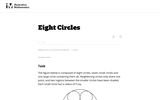Unrestricted Use
CC BY
Rating
0.0 stars
Overview:

The purpose of this task is to strengthen students' understanding of area. It could be assigned in class to individuals or small groups or given as a homework exercise to generate interesting discussions the following day. The relatively high levels of complexity and technical demand enhance its instructional value.

Subject:
Mathematics
Material Type:
Activity/Lab
Provider:
Illustrative Mathematics
Provider Set:
Illustrative Mathematics
Author:
Illustrative Mathematics
Date Added:
05/01/2012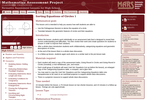Only Sharing Permitted
CC BY-NC-ND
Rating
4.5 stars
Overview:

This lesson unit is intended to help teachers assess how well students are able to: use the Pythagorean theorem to derive the equation of a circle; and translate between the geometric features of circles and their equations.

Subject:
Geometry
Material Type:
Assessment
Lesson Plan
Provider:
Shell Center for Mathematical Education
Provider Set:
Mathematics Assessment Project (MAP)
Date Added:
07/31/2019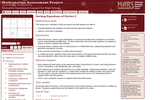Only Sharing Permitted
CC BY-NC-ND
Rating
4.0 stars
Overview:

This lesson unit is intended to help teachers assess how well students are able to: translate between the equations of circles and their geometric features; and sketch a circle from its equation.

Subject:
Geometry
Material Type:
Assessment
Lesson Plan
Provider:
Shell Center for Mathematical Education
Provider Set:
Mathematics Assessment Project (MAP)
Date Added:
07/31/2019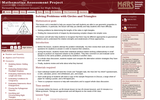Only Sharing Permitted
CC BY-NC-ND
Rating
5.0 stars
Overview:

This lesson unit is intended to help teachers assess how well students are able to use geometric properties to solve problems. In particular, the lesson will help you identify and help students who have the following difficulties: solving problems by determining the lengths of the sides in right triangles; and finding the measurements of shapes by decomposing complex shapes into simpler ones. The lesson unit will also help students to recognize that there may be different approaches to geometrical problems, and to understand the relative strengths and weaknesses of those approaches.

Subject:
Geometry
Material Type:
Assessment
Lesson Plan
Provider:
Shell Center for Mathematical Education
Provider Set:
Mathematics Assessment Project (MAP)
Date Added:
06/24/2019Conditional Remix & Share Permitted
CC BY-NC-SA
Rating
0.0 stars
Overview:

CK-12's Texas Instruments Geometry Student Edition Flexbook is a useful collection of exercises intended to enrich a student's understanding of basic geometric principles.

Subject:
Mathematics
Material Type:
Textbook
Provider:
CK-12 Foundation
Provider Set:
CK-12 FlexBook
Author:
Jordan, Lori
Date Added:
12/14/2010Read the Fine Print
Rating
0.0 stars
Overview:

In this lesson, students will duplicate the method Archimedes used to estimate the value of pi.

Subject:
Mathematics
Math 1
Material Type:
Activity/Lab
Lesson Plan
Provider:
PBS
Author:
Nova Teachers
Date Added:
02/26/2019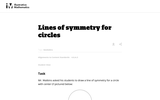Unrestricted Use
CC BY
Rating
0.0 stars
Overview:

This is an instructional task that gives students a chance to reason about lines of symmetry and discover that a circle has an an infinite number of lines of symmetry. Even though the concept of an infinite number of lines is fairly abstract, fourth graders can understand infinity in an informal way.

Subject:
Mathematics
Material Type:
Activity/Lab
Provider:
Illustrative Mathematics
Provider Set:
Illustrative Mathematics
Author:
Illustrative Mathematics
Date Added:
11/11/2012Read the Fine Print
Rating
0.0 stars
Overview:

This classroom activity, which centers on documenting the location of a meteorite in Antarctica, introduces students to the technology of Global Positioning Systems. The printable handout includes a series of inquiry-based questions to get students thinking about the challenges of marking exact positions in an area where the landscape is continually changing, illustrated activity directions and a worksheet that guides students through the way satellites are used in GPS and includes areas for recording their findings.

Subject:
Science
Material Type:
Activity/Lab
Diagram/Illustration
Lesson Plan
Simulation
Provider:
American Museum of Natural History
Author:
American Museum of Natural History
Rice University
Date Added:
07/31/2019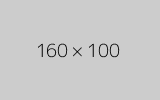Conditional Remix & Share Permitted
CC BY-NC
Rating
0.0 stars
Overview:

Four full-year digital course, built from the ground up and fully-aligned to the Common Core State Standards, for 7th grade Mathematics. Created using research-based approaches to teaching and learning, the Open Access Common Core Course for Mathematics is designed with student-centered learning in mind, including activities for students to develop valuable 21st century skills and academic mindset.

Subject:
Mathematics
Material Type:
Full Course
Provider:
Pearson
Date Added:
11/02/2020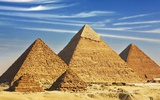Rating
0.0 stars
Overview:

Zooming In On Figures

Unit Overview

Type of Unit: Concept; Project

Length of Unit: 18 days and 5 days for project

Prior Knowledge

Students should be able to:

Find the area of triangles and special quadrilaterals.
Use nets composed of triangles and rectangles in order to find the surface area of solids.
Find the volume of right rectangular prisms.
Solve proportions.

Lesson Flow

After an initial exploratory lesson that gets students thinking in general about geometry and its application in real-world contexts, the unit is divided into two concept development sections: the first focuses on two-dimensional (2-D) figures and measures, and the second looks at three-dimensional (3-D) figures and measures.
The first set of conceptual lessons looks at 2-D figures and area and length calculations. Students explore finding the area of polygons by deconstructing them into known figures. This exploration will lead to looking at regular polygons and deriving a general formula. The general formula for polygons leads to the formula for the area of a circle. Students will also investigate the ratio of circumference to diameter ( pi ). All of this will be applied toward looking at scale and the way that length and area are affected. All the lessons noted above will feature examples of real-world contexts.
The second set of conceptual development lessons focuses on 3-D figures and surface area and volume calculations. Students will revisit nets to arrive at a general formula for finding the surface area of any right prism. Students will extend their knowledge of area of polygons to surface area calculations as well as a general formula for the volume of any right prism. Students will explore the 3-D surface that results from a plane slicing through a rectangular prism or pyramid. Students will also explore 3-D figures composed of cubes, finding the surface area and volume by looking at 3-D views.
The unit ends with a unit examination and project presentations.

Subject:
Mathematics
Geometry
Provider:
PearsonConditional Remix & Share Permitted
CC BY-NC
Rating
0.0 stars
Overview:

Lesson OverviewStudents will compare the formula for the area of a regular polygon to discover the formula for the area of a circle.Key ConceptsThe area of a regular polygon can be found by multiplying the apothem by half of the perimeter. If a circle is thought of as a regular polygon with many sides, the formula can be applied.For a circle, the apothem is the radius, and p is C.A=a(p2)→A=rC2→A=rπd2→A=rπ2r2→A=rπr=πr2 GoalsDerive the formula for the area of a circle.Apply the formula to find the area of circles.SWD: Consider the prerequisite skills for this lesson: understanding and applying the formula for the area of a regular polygon. Students with disabilities may need direct instruction and guided practice with this skill.Students should understand these domain-specific terms:apothemparallelogramderivationheightapproximate (estimate)scatter plotpiperimetercircumferenceIt may be helpful to preteach these terms to students with disabilities.

Subject:
Geometry
Material Type:
Lesson Plan
Author:
Pearson
Date Added:
11/02/2020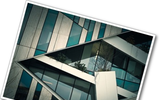Conditional Remix & Share Permitted
CC BY-NC
Rating
0.0 stars
Overview:

Gallery OverviewAllow students who have a clear understanding of the content thus far in the unit to work on Gallery problems of their choosing. You can then use this time to provide additional help to students who need review of the unit’s concepts or to assist students who may have fallen behind on work.Problem DescriptionsSprinklersExplore different sprinkler layouts, looking at circular areas (and partial circles) to decide which will be best to water a lawn.Leaning TowerChoose a scale and use a ruler and protractor to make a simple scale drawing.Pizza DoublerIf you could choose between doubling the fraction of the pizza that a slice is, or doubling the radius, which option would give you more pizza? In this problem you will investigate which choice gives a bigger slice.Area and ScaleWhen a figure is redrawn at a larger scale the side lengths increase by the factor of the scale (if the scale doubles the size, the side lengths double also). But, does the area increase the same way? Explore a dynamic sketch and see how area changes when the scale changes.Tree House 1Given plans for a tree house, redraw the plans at a different scale.

Subject:
Geometry
Material Type:
Lesson Plan
Author:
Pearson
Date Added:
11/02/2020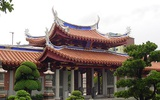Conditional Remix & Share Permitted
CC BY-NC
Rating
0.0 stars
Overview:

Students critique their work from the Self Check and redo the task after receiving feedback. Students then take a quiz to review the goals of the unit.Key ConceptsStudents understand how to find the area of figures such as rectangles and triangles. They have applied that knowledge to finding the area of composite figures and regular polygons. The area of regular polygons was extended to understand the area of a circle. Students also applied ratio and proportion to interpret scale drawings and redraw them at a different scale.GoalsCritique and revise student work.Apply skills learned in the unit.Understand two-dimensional measurements:Area of composite figures, including regular polygons.Area and circumference of circles.Interpret scale drawings and redraw them at a different scale.SWD: Make sure all students have the prerequisite skills for the activities in this lesson.Students should understand these domain-specific terms:composite figuresregular polygonsareacircumferencescale drawingstwo dimensionalIt may be helpful to preteach these terms to students with disabilities.ELL: As academic vocabulary is reviewed, be sure to repeat it and allow students to repeat after you as needed. Consider writing the words as they are being reviewed. Allow enough time for ELLs to check their dictionaries if they wish.

Subject:
Geometry
Material Type:
Lesson Plan
Author:
Pearson
Date Added:
11/02/2020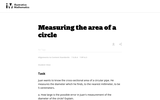Unrestricted Use
CC BY
Rating
4.0 stars
Overview:

This goal of this task is to give students familiarity using the formula for the area of a circle while also addressing measurement error.

Subject:
Mathematics
Material Type:
Activity/Lab
Provider:
Illustrative Mathematics
Provider Set:
Illustrative Mathematics
Author:
Illustrative Mathematics
Date Added:
10/12/2012
Remix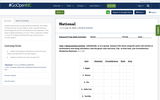Conditional Remix & Share Permitted
CC BY-NC
Rating
0.0 stars
Overview:

Students will practice measuring the diameter and radius of food themed circular objects. Next, they will discover that finding the ratio of circumference and diameter is the value of approximately 3.14 or pi.
Then, using the number 3.14 for Pi, students will find the area of the various circular objects.

Subject:
Algebra
Geometry
Math 1
Material Type:
Activity/Lab
Homework/Assignment
Author:
PATRICIA NORTON
Date Added:
07/10/2020
Remix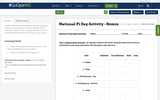Conditional Remix & Share Permitted
CC BY-NC
Rating
0.0 stars
Overview:

Students will practice measuring the diameter and radius of various circular objects. Next, they will discover the ratio of pi to about 3.
Then, using the number 3.14 for Pi, students will find the area of the various circular objects.

Subject:
Algebra
Geometry
Math 1
Material Type:
Activity/Lab
Homework/Assignment
Author:
CHERYL WILLIAMS
Date Added:
11/06/2019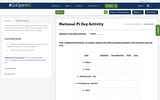Conditional Remix & Share Permitted
CC BY-NC
Rating
0.0 stars
Overview:

Students will practice measuring the diameter and radius of various circular objects. Then using the number 3.14 for Pi, will find the area of the various circular objects.

Subject:
Algebra
Geometry
Math 1
Material Type:
Activity/Lab
Homework/Assignment
Author:
Nathan Rutko
Date Added:
11/06/2019
Remix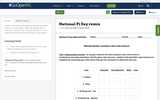Conditional Remix & Share Permitted
CC BY-NC
Rating
0.0 stars
Overview:

Students will practice measuring the diameter and radius of various circular objects. Next, they will discover the ratio of pi to about 3.
Then, using the number 3.14 for Pi, students will find the area of the various circular objects.

Subject:
Algebra
Geometry
Math 1
Material Type:
Activity/Lab
Homework/Assignment
Author:
Nathan Rutko
Date Added:
07/07/2020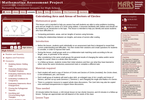Only Sharing Permitted
CC BY-NC-ND
Rating
4.0 stars
Overview:

This lesson unit is intended to help teachers assess how well students are able to solve problems involving area and arc length of a sector of a circle using radians. It assumes familiarity with radians and should not be treated as an introduction to the topic. This lesson is intended to help teachers identify and assist students who have difficulties in: Computing perimeters, areas, and arc lengths of sectors using formulas and finding the relationships between arc lengths, and areas of sectors after scaling.

Subject:
Geometry
Material Type:
Assessment
Lesson Plan
Provider:
Shell Center for Mathematical Education
Provider Set:
Mathematics Assessment Project (MAP)
Date Added:
07/31/2019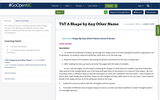Conditional Remix & Share Permitted
CC BY-NC-SA
Rating
3.0 stars
Overview:

This resource is part of Tools4NCTeachers.

After reading the story, The Silly Story of Goldilocks and the Three Squares, students identify and describe shapes in a variety of orientations.

Remix this lesson to your your extension ideas!

Subject:
Mathematics
Material Type:
Lesson
Lesson Plan
Author:
DAWNE COKER
Date Added:
11/06/2019